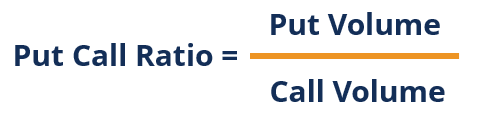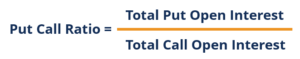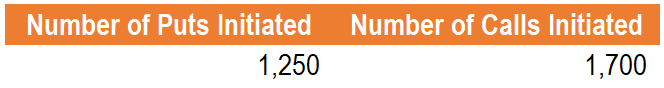# Put-Call Ratio (PCR)

A ratio that uses the volume of puts and calls over a determined time period on a market index to determine market sentiment

## What is the Put-Call Ratio (PCR)?

The put-call ratio (PCR) is an indicator used by investors to gauge the outlook of the market. The ratio uses the volume of puts and calls over a determined time period on a market index to determine market sentiment. It can additionally be used for individual securities by looking at the volume of puts and calls on a security over a determined time period. A high PCR is indicative of bearish sentiment while a low PCR is indicative of bullish sentiment.

### Summary:

• The put-call ratio (PCR) is an indicator used by investors to gauge the outlook of the market.
• The PCR is calculated as put volume over a determined time period dividend by call volume over the same time period.
• The ratio is interpreted differently depending on the type of investor.

### Formula for the Put-Call Ratio

The formula for the put-call ratio is as follows:Where:

• Put Volume is the number of put options initiated over a determined time period; and
• Call Volume is the number of call options initiated over the same time period.

It is important to note that the PCR is not limited to put volume and call volume in its calculation. It is common for investors to use a variation, as shown below:Where:

• Total Put Open Interest is the total number of outstanding puts; and
• Total Call Open Interest is the total number of outstanding calls.

### Example of the Put-Call Ratio

An investor is looking to use the put-call ratio as a preliminary measure of sentiment on a security. The security showed the following puts and calls initiated over the more recent trading day:The put-call ratio for the security is 1,250 / 1,700 = 0.7353.

### How to Interpret the Put-Call Ratio

#### 1. Interpreting the Number

A PCR below one (<1) suggests that investors are purchasing more call options than put options and signals that investors are speculating a bullish trend going forward.

A PCR above one (>1) suggests that investors are purchasing more put options than call options and signals that investors are speculating a bearish trend going forward.

A PCR at one (=1) suggests that investors are purchasing the same amount of put options as call options and signals a neutral trend going forward.

No PCR is considered ideal, but a PCR  below 0.7 is typically viewed as a strong bullish sentiment while a PCR above 1 is typically viewed as a strong bearish sentiment.

#### 2. Investment Style

It is important to note that the PCR is interpreted differently depending on the investor’s investment style. For example, consider two investment styles:

A contrarian investor may consider a PCR as a bullish signal while a low PCR as a bearish signal.

A momentum investor may consider a high PCR as a bearish signal while a low PCR as a bullish signal.

Therefore, the PCR depends on the investor’s investment style, and, as such, there is no one correct way to interpret the ratio.

### Put-Call Ratio in the Market

A popular website used to track the put-call ratio in the market is cboe.com. Cboe provides the PCR for a number of indexes and products. As of July 12, 2019, the index PCR was 1.15, and the exchange-traded funds PCR was 1.32.